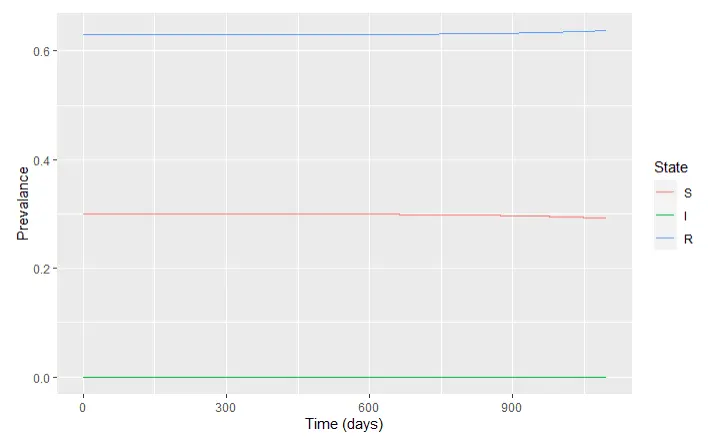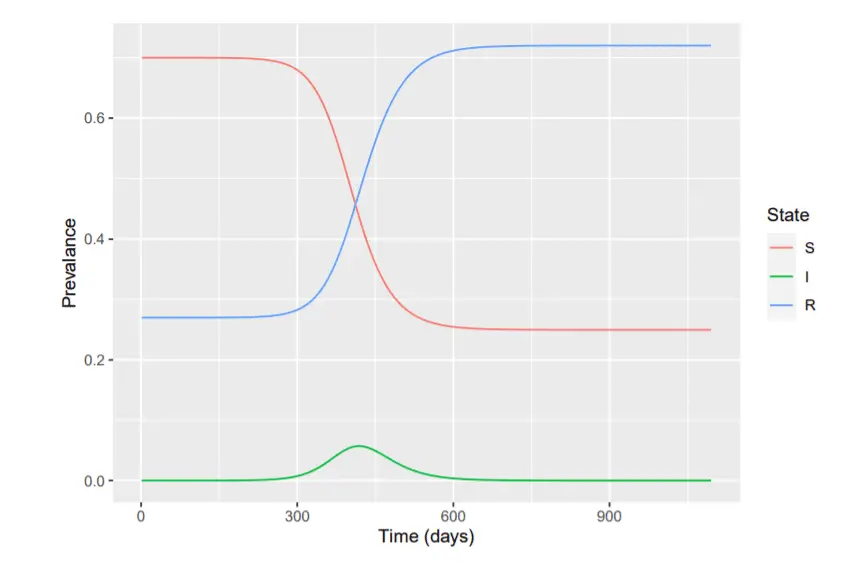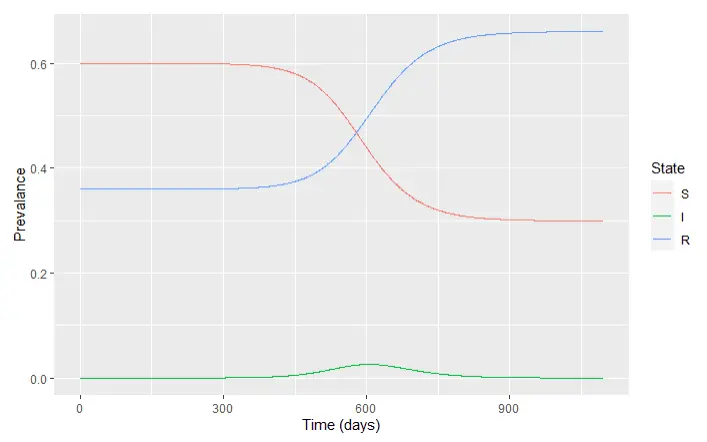# Team:UCDavis/Disease Modeling

Disease Modeling | iGEM Project Cargo

# Disease Modeling

Using a modified SIR model in collaboration with Team UCSC to model disease spread

by Ethan Wright

# How to Track a Pandemic

Any effective public health initiative is based on modeling and previous data. The spread of disease in a population and how it is affected by vaccination is a multifactor system. Some would assume that it would only be affected by the number of people who get vaccinated, but also how soon they get vaccinated, the efficacy of the vaccine, and many other variables. The sooner someone gets vaccinated, the sooner a population achieves herd immunity. This is important for the evolution of virus strains. The longer a population takes to achieve herd immunity, the more time it has to spread, replicate, and mutate into strains that current vaccines in roll out are not able to protect against.

To demonstrate the influence of vaccination amount, timing, efficacy, and strain evolution we modified a standard SIR model to better illustrate the effect of many different factors on when we reach herd immunity.

# SIR Model

The SIR model is the basis for infectious disease modelling. It is a population based compartmental model where individuals belong to one of three compartments: Susceptible (S), Infectious (I), and Recovered (R). The change in each compartment over time is based on a set of differential equations with infection rate and recovery rate coefficients.

$$\frac{dS}{dt} = -\beta S \frac{1}{N}$$ $$\frac{dI}{dt} = \beta S \frac{1}{N} -\gamma I$$ $$\frac{dR}{dt} = \gamma I$$

is the infection rate, is the infection rate, N is the total population, S is the susceptible population,I is the infected population, and R is the recovered population.

To extend our model to include vaccination effects we consulted with the UC Santa Cruz iGEM team. They taught us how to properly alter the differential equations to include the vaccination compartment. Working with UC Santa Cruz we have added a variable p that will represent the proportion of the population vaccinated and a coefficient ve to represent vaccine efficacy. These two factors will change the initial conditions to reflect the vaccinated population.

The first SIR model illustrates the percentage of the population in each compartment over a three year period. This example is with 30% of the population vaccinated and a 90% effective vaccine. We can see the green line representing infection rate peaks around 450 days with a peak of 5% of the population infected.The second model is with 40% of the population vaccinated and we can see that the infection peak is much milder and severely delayed peaking around 2.5% of the population and 600 days. The final model is a population with 70% of the population vaccinated and we can see that all three curves are flat. This means that the population has achieved herd immunity and will not suffer an outbreak. These three models illustrate the advantages of early vaccination as with higher vaccination rates we can reduce infection peaks and reach herd immunity much more quickly.Figure 1: Population over 3 yearsFigure 2: 40% of population vaccinatedFigure 3: 70% of population vaccinated